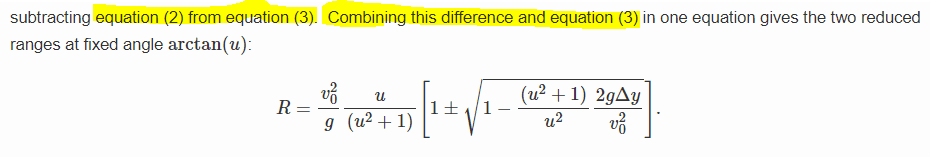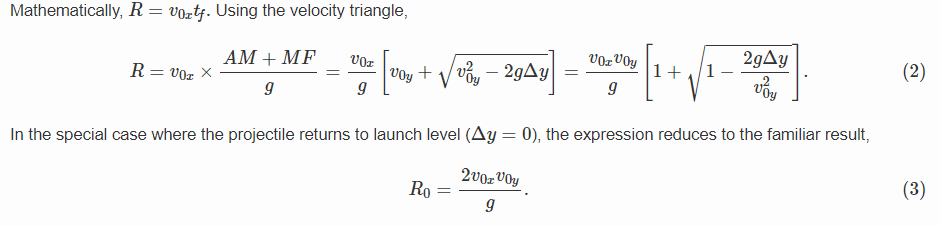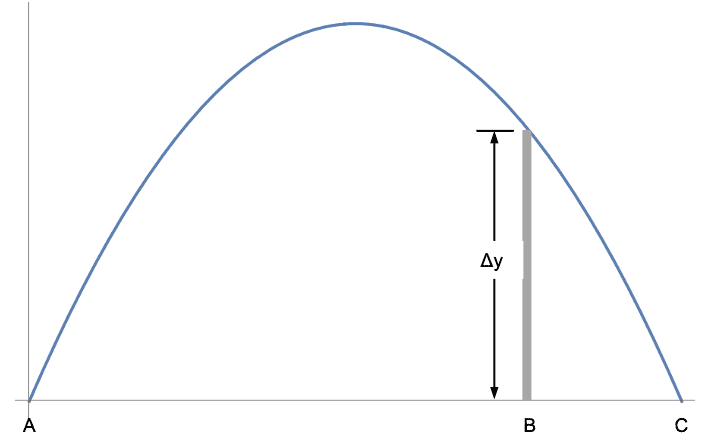Homework Helper
Gold Member
Introduction
In a homework thread a while back a PF member expressed dismay along the lines of “oh no, not another boring projectile motion problem.” Admittedly, I shared the member’s sentiments at the time.  Yet after some thought, I concluded that it is the unvarying recommended strategy of the genre that makes it boring: (a) Write down the  SUVAT equations ada[ted for projectile motion; (b) find the time of flight from the vertical motion equation; (c) use the time of flight to find whatever else you need. “Perhaps,” I thought, “relief from ennui might be found if one forsakes the traditional approach altogether.  How about a formulation of projectile motion based on geometry and trigonometry that allows analysis without quadratic equations?” This tutorial is a streamlined account of how to do it.
The velocity triangle
In 2-d projectile motion without air resistance the equation relating the velocity ##\vec v_{\!f}## at time ##t_{\!f}## and the initial velocity ##\vec v_0##  is...

Last edited:
•neilparker62, Yashbhatt, etotheipi and 1 other person

Homework Helper
2022 Award
An interesting method. Two quick comments:
1) I find the sentence immediately following eqn. 1 to be confusing (and unnecessary unless I am missing something)
2) I am a little bit worried pedagogically about yet another method where vectors are represented on paper. In my experience I am happy if students at this stage can, with facility, simply add and subtract multiple vectors using a graphical representation, head to toe. Maybe I am projecting here...I remember it was difficult until I "got" it

Homework Helper
Gold Member
An interesting method. Two quick comments:
1) I find the sentence immediately following eqn. 1 to be confusing (and unnecessary unless I am missing something)
2) I am a little bit worried pedagogically about yet another method where vectors are represented on paper. In my experience I am happy if students at this stage can, with facility, simply add and subtract multiple vectors using a graphical representation, head to toe. Maybe I am projecting here...I remember it was difficult until I "got" it

1. I edited the sentence in question to read, "When the projectile drops below the launch point, the vertical displacement ##\Delta y## (a signed quantity) is negative therefore ##(-2g\Delta y)## in the equation must be replaced with ##(2g |\Delta y|)##." I believe that this is an important statement because it shows that all the equations developed here are applicable regardless of where the projectile lands relative to the launch level.

2. I agree with you that yet another method for analyzing projectile motion is pedagogically worrisome. Projectile motion is introduced immediately after 1-d kinematics as an illustration of how the SUVAT equations can be extended to two dimensions. Abandoning the continuity from 1-d to 2-d at this point and talking about velocity triangles would be a disservice to the students. I see velocity triangles used by instructors when students, who have already (more or less) mastered the use of the horizontal and vertical kinematic equations, try to make sense out of answers they have obtained algebraically. "Here is another way of looking at this" is pedagogically sounder than "it makes sense because it came out of the equations and the algebra is correct." This insight is intended as a possible addition to an instructor's toolbox.

•hutchphd
Leo Liu
Hi. Thank you for your insight.
I just have a small question about finding the range of the projectile flying over a slide--why did you add the difference to eq 3 rather than eq2? I would like to know this because I think R_0 is already the range of the projectile which returns to the starting height, and it makes no sense if you add the difference to it. Thanks.----------------------------------------------------------------------------------------------------------------------------------------------------------Homework Helper
Gold Member
Hi. Thank you for your insight.
I just have a small question about finding the range of the projectile flying over a slide--why did you add the difference to eq 3 rather than eq2? I would like to know this because I think R_0 is already the range of the projectile which returns to the starting height, and it makes no sense if you add the difference to it. Thanks.
View attachment 273653
----------------------------------------------------------------------------------------------------------------------------------------------------------
View attachment 273654
I did not add any difference.

Equation (3) gives ##R_0## and is represented by length AC in the figure below.
Equation (2) gives the horizontal distance to where the projectile is at height ##\Delta y## and is represented by length AB in the figure below.
When you subtract equation (2) from equation (3), you get the remainder of ##R_0## which is the "other" range represented by length BC in the figure below.

I wrote the two ranges in one equation that gives either ##R##. The top (positive) sign is for the longer AB and the bottom (negative) sign is for the shorter BC. That same equation with ##\Delta y =0## gives ##R_0.##•Leo Liu
Homework Helper
Re maximum range when ##v_0## is perpendicular ##v_f##. Seems reasonable but are we assuming that based on intuition or is there a proof ? Perhaps something along following lines:

https://math.stackexchange.com/questions/2660468/projectile-vw-gk-for-minimum-launch-velocity/2687554#2687554

I am presuming that minimum launch velocity for a given range is a similar problem to maximum range for a given launch velocity.

Homework Helper
"Equally unused, untaught and apparently not even assigned as a “show that” exercise is Equation (4) that identifies the range as the magnitude of the cross product of the initial and final velocity divided by g. It appears that this beautiful equation has been ignored because of adherence to the quadratic formulation as the only method for addressing problems in projectile motion."

Equation 4 is brilliant! I have used it to solve a whole host of 2D projectile problems. For example all of the problems in this set except the last two on centripetal force.

https://www.kpu.ca/sites/default/files/Faculty%20of%20Science%20&amp;%20Horticulture/Physics/PHYS%201120%202D%20Kinematics%20Solutions.pdf

Recommend readers try it out !

Last edited:
Homework Helper
Gold Member
"Equally unused, untaught and apparently not even assigned as a “show that” exercise is Equation (4) that identifies the range as the magnitude of the cross product of the initial and final velocity divided by g. It appears that this beautiful equation has been ignored because of adherence to the quadratic formulation as the only method for addressing problems in projectile motion."

Equation 4 is brilliant! I have used it to solve a whole host of 2D projectile problems. For example all of the problems in this set except the last two on centripetal force.

https://www.kpu.ca/sites/default/files/Faculty of Science &amp; Horticulture/Physics/PHYS 1120 2D Kinematics Solutions.pdf

Recommend readers try it out !
This link appears to be broken.

Homework Helper
This link appears to be broken.
Seems ok from here ? I'm not sure why that should be different for you but it's not the first time certain links seem to be accessible to some but not others ??